### Stress: Engineering vs True Stress for Metals

#### Learning Outcomes

• Differentiate between the engineering and the true stress
• Compute the “true stress vs. true strain” curve of a metal given the “engineering stress vs. engineering strain” curve.

### Engineering vs True Stress

During material uniaxial tests, the value of the applied stress is obtained by dividing the applied force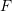by the measured initial cross sectional area of the specimen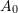. In this case, the stress is termed the “Engineering Stress”. If excessive decrease (or increase) in the cross sectional area occurs, then the force should be divided by the actual area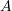(area after deformation) to obtain the “True Stress”: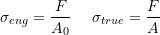Similarly, the “Engineering Strain” as defined in uniaxial strain measures is obtained by dividing the elongation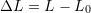by the original length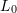, while the “True Strain” is obtained by taking the natural logarithm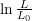: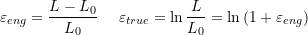Considering the behaviour of metals, at higher strains, the deformation of a metal is volume preserving and thus: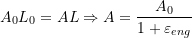In this case, the true stress is related to the engineering stress as follows: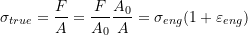#### Example

The engineering stress vs. the engineering strain of a metal is given by the following table:

Compute the true stress and the true strain. Plot the engineering and the true stress vs. strain relationships.

##### SOLUTION:

The following table is constructed using the equations for the true stress and true strain: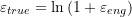As shown in the plot above, the values of the true stress for metals are usually slightly higher than the values of the engineering stress for strains higher than 0.005.